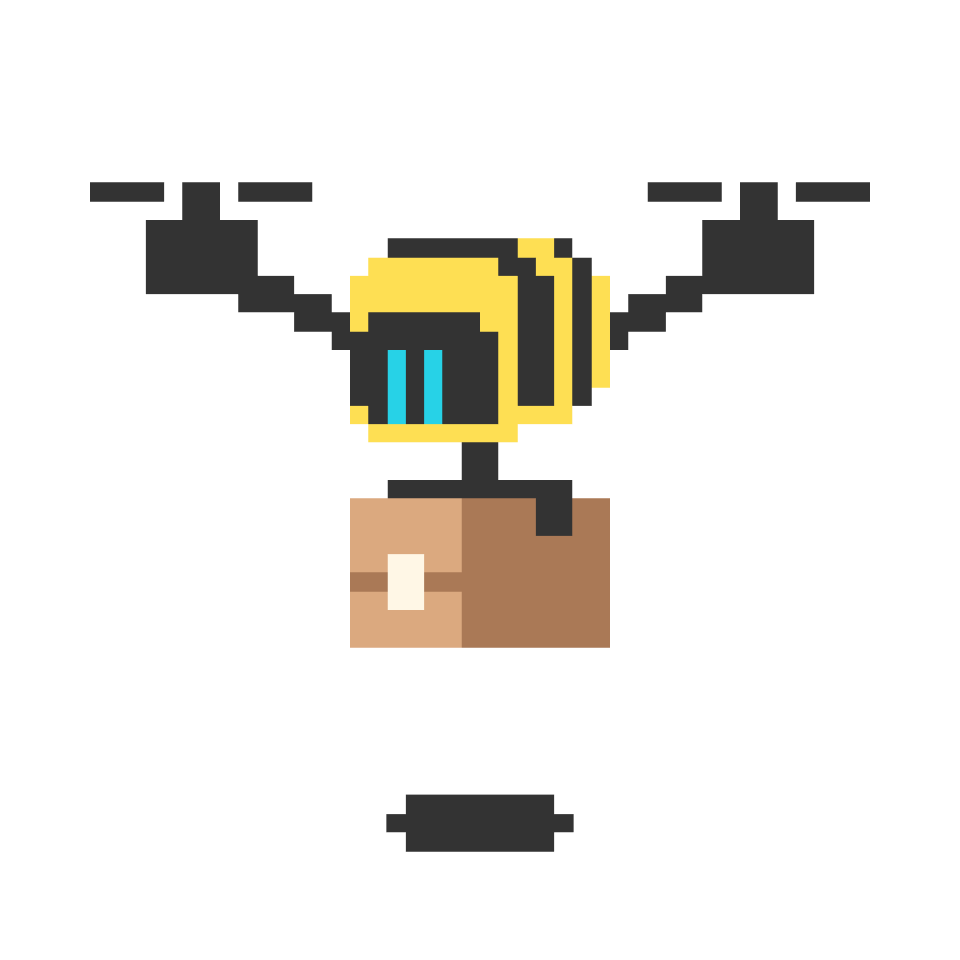# Thinking with Graphs

Can you walk across each of the seven bridges of Königsberg exactly once? (You cannot. But the interesting part is explaining why you cannot!)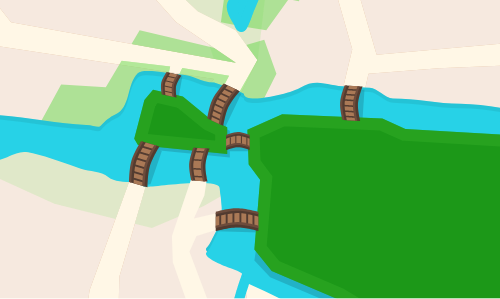You previous explored the possibility of a brute force solution: checking every permutation of bridges. Like other brute force solutions, it only well for small numbers of bridges

It's possible to make progress, and figure out how to solve the problem for cities with many more bridges, by thinking about the problem in a more general way.

## Thinking with Graphs

### Tools of Computer Science

# Thinking with Graphs

The shape of the landmasses in Königsberg never mattered—you can walk from any place on the northern shore to any other place without crossing a bridge. You can therefore represent these four landmasses as four points. Because the islands are to the east and west, and the banks are to the north and south, label these four landmasses N, S, E, and W.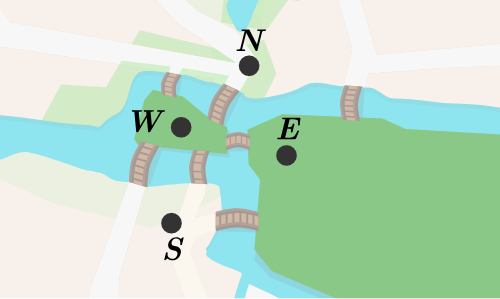The position and shape of the bridges also don't matter. Replace the bridges with curves connecting the points: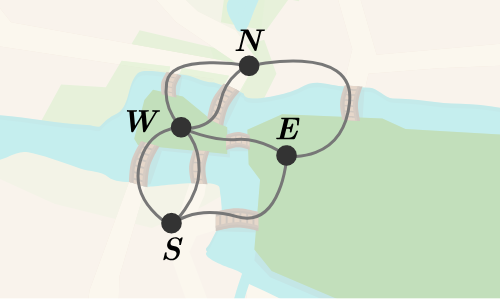You now have a structure called a graph. A mathematician or computer scientist would say that the points we labeled N, S, E, and W are vertices and that the curves connecting them (which represent the bridges) are edges.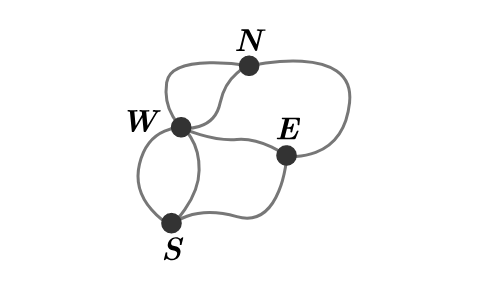## Thinking with Graphs

### Tools of Computer Science

# Thinking with Graphs

Computer scientists care a lot about graphs. Not the kind of graphs that have X- and Y-axes, though those are nice, too—computer scientists are very interested in graphs made up of vertices connected by edges.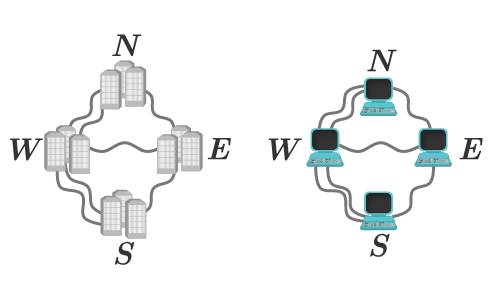Lots of important things can be represented with graphs! Someone interested in electrical grids might represent cities as vertices and the power lines connecting them as edges. Someone interested in computer networks might represent computers as vertices and the network connections between them as edges.

If you were a computer scientist working for a city government, which of these graphs might be interesting to you? (Select all that apply.)

## Thinking with Graphs

### Tools of Computer Science

Select one or more

# Thinking with Graphs

Here's the graph representing the bridges of Königsberg. There are four vertices and seven edges.How many of the vertices have an odd number of connected edges?

## Thinking with Graphs

### Tools of Computer Science

# Thinking with Graphs

Using graphs, it's time to rephrase the puzzle of the seven bridges of Königsberg:

Can you travel from vertex to vertex in a graph along edges, visiting each edge exactly once?

A path through a graph that visits every edge once is called a Eulerian path. Eulerian paths are named after Leonhard Euler, who is credited with first solving the puzzle of the seven bridges of Königsberg.

This animation shows 2 failed attempts at finding a Eulerian path in a graph and 1 successful one. The Eulerian path is the one that uses every edge exactly once. It doesn't matter how many times a vertex is passed.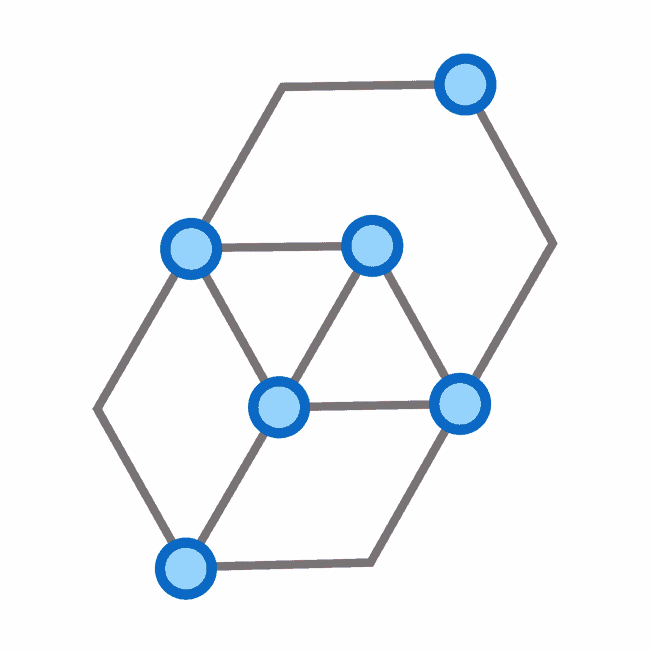## Thinking with Graphs

### Tools of Computer Science

# Thinking with Graphs

Keeping in mind that a path through a graph that visits every edge once is called a Eulerian path, take a look at what happens whenever any path goes through a vertex.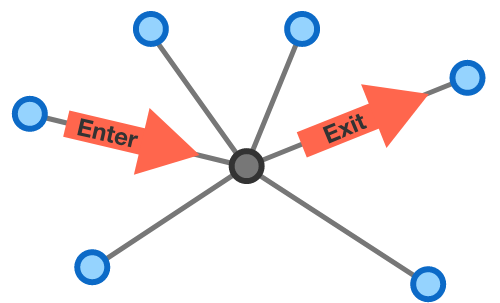No matter how many edges are connected to a vertex, when your path passes through a vertex, you "use up" two of its connected edges: one edge is touched when you enter, and another is touched when you leave.

Imagine you have a graph that does have a Eulerian path. One of the vertices has an odd number of connected edges. What do you know about this vertex?

## Thinking with Graphs

### Tools of Computer Science

# Thinking with Graphs

You've just learned two facts:

• If any one vertex has an odd number of connected edges, it must be at the beginning or the end of a Eulerian path. (This is a fact about graphs, and is true for any graph.)
• The graph representing the bridges of Königsberg has four vertices with an odd number of connected edges. (This is just a fact about the city of Königsberg, specifically.)

A path only has one end and one beginning, so there's no way for a single path to start and end at four different places. This means that the bridges of Königsberg cannot all be crossed exactly once!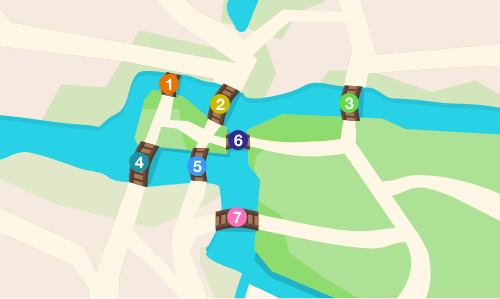Which of these bridges, if removed (leaving the other six bridges), would allow there to be a Eulerian path?

## Thinking with Graphs

### Tools of Computer Science

# Thinking with Graphs

You've learned a new fact about graphs: if there are more than two vertices with an odd number of connected edges, there can't be a Eulerian path—that is, a path that uses every edge exactly once.

That new fact can be applied to other graphs.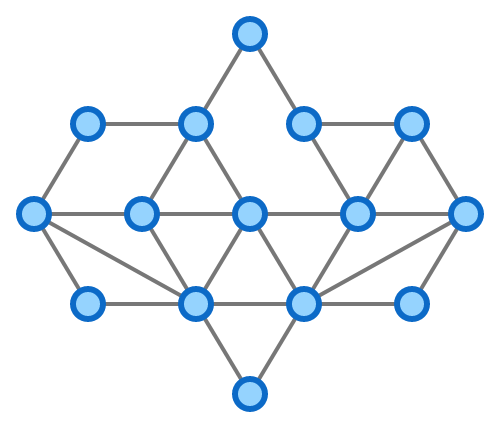Is there a Eulerian path in this graph?

## Thinking with Graphs

### Tools of Computer Science

# Thinking with Graphs

We've made our problem about the seven bridges of Königsberg more general by turning it to a problem about graphs. Our graph understanding lets us understand other graphs. It can also help us understand other concrete problems that can be rephrased as graph problems!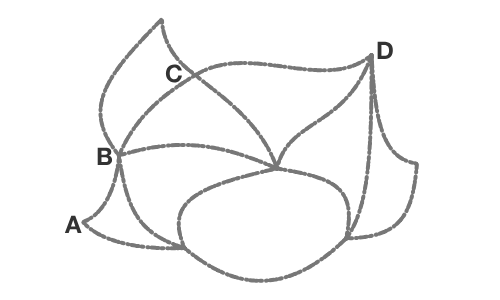Boris challenges Anke to draw this sketch on paper without lifting her pencil and without retracing any part of the sketch. Which of the labeled points should Anke start drawing from?

## Thinking with Graphs

### Tools of Computer Science

# Thinking with Graphs

In this quiz, you reinterpreted the classic mathematical puzzle of the seven bridges of Königsberg as a graph problem, much as Leonhard Euler did in the eighteenth century.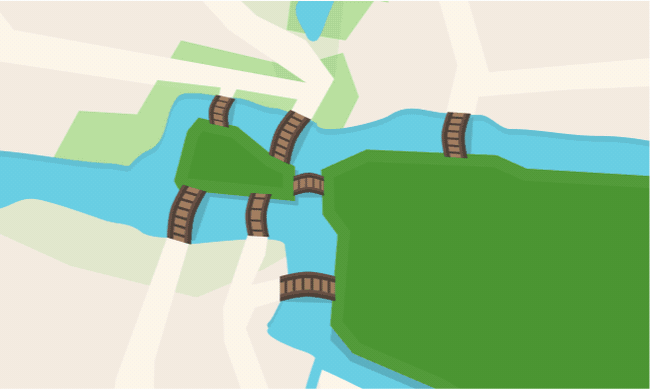This approach let you avoid using the slow brute force approach altogether. That's an example of the real power and magic of computer science: taking an intractable problem and making it tractable by understanding its structure.

Even better, when you solve a concrete problem (like the puzzle of the seven bridges of Königsberg) by understanding a more general problem (like Eulerian paths in graphs), you can apply that general understanding to other problems.

This exploration only looked at a few simple puzzles, but understanding problems with the help of graphs is an incredibly important tool for computer scientists.

## Thinking with Graphs

### Tools of Computer Science

×

Problem Loading...

Note Loading...

Set Loading...